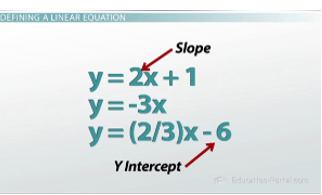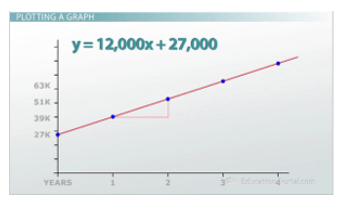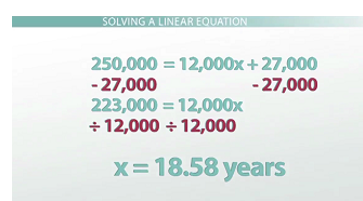# What is a Linear Equation?

#### Video Lesson on What is a Linear Equation?

This lesson video Not available at this time available video coming soon

### What is a Linear Equation?

Most cars won't be able to run for more than 250,000 miles, so how much longer will your car live? Linear Equations are the most basic kind of algebraic function and can help you answer questions exactly like this. Learn about what they look like, how they come up in your life and why they are powerful tools.

### Defining a Linear Equation

This lesson is on what a linear equation is. And the answer to that question is essentially a linear equationis any pattern of numbers that is increasing or decreasing by the same amount every step of the way. This means that the only two things that we need to define a linear equation are where the pattern begins and what that pattern moves by. What that leaves us with is the slope-intercept form of the linear equation, y = mx + b, where the m value is the slope, and the b value is the y-intercept.Examples of linear equations in slope-intercept form

Some examples of linear equations in slope-intercept form: You could have y = 2x + 1; you could have y = -3x; and you could have y = (2/3)x - 6. In each equation, the number in front of the x represents the slope, or the number that it's moving by. The number on the end represents where it begins, or the y-intercept. If there is no number after the x, that implies that the y-intercept is zero.

### Building a Linear Equation

What's the point? Why do I care about a linear equation? Well, linear equations are the most common patterns of numbers we see, and they can be used to describe all sorts of situations you see around you.

One example has to do with the first car I bought. As soon as I turned 16, my parents helped me buy a used car. When I got my car it had 27,000 miles on it, and I've owned it for a while now. Every year, I drive it about 12,000 more miles. What we end up with is a linear equation to represent the situation that looks like y = 12,000x + 27,000, because I drive it 12,000 more miles every year, and that's how much the pattern moves by. And the pattern began at 27,000 miles when I first bought the car. So, after year one, it had 39,000; after year two, it had 51,000; and after year three, it had 63,000. And so on.

### Plotting a Graph

Drawing a graph of this linear equation can help us make a lot of predictions about what this pattern will do in the future, and it can also give us a better sense of the pattern that may not be easy to see just from looking at the numbers. When I want to graph a linear equation, I use the y-intercept, the b value as the beginning value on my graph. So, before I had driven the car a single day, it already had 27,000 miles on it because I got it used. After year one, I'd been driving it along, and after that year I was up to 39,000 miles. I kept driving it and driving it, so after year two I was up to 51,000 miles. So, every time I go over one year, I go up by 12,000 miles.

What we end up with are a bunch of points that are exactly in a row, and these points form a line. All linear equations come across as lines when you graph them - which makes sense, since linear and line are almost the same word. While I was drawing the graph, you may have noticed the triangle that I drew underneath where I went over one year and up 12,000 miles. This triangle is something called a slope triangle, and it helps us determine and draw the slope of the graph. Again, the slope is another name for how much the pattern is moving by. The slope is also what we call the rise over the run; that basically means how it goes up and down divided by how much it goes left and right.Graph of a linear equation

This graph is really nice because it tells me how many miles will be on my car at any one point in just one picture, but these are really only estimates. If I wanted to know how many miles were on my car after 1 year, 7 months and 6 days, I'd have to go up to this point and read it over. We could guess that it's around 50, but we don't really know. And that's where the algebra comes in to it.

### Solving a Linear Equation

So, it's my understanding that most cars last around 250,000 miles; I think I'd be happy if my car made it that far. My question is how much longer do I have? Will my car last as long as I think it will, or am I going to have to get another car pretty soon? We can use the equation to answer that question, where y is the number of miles and x is the number of years that have gone by: 250,000 = 12,000x + 27,000.

At this point I can undo the things that have been done to x to isolate x and get the number of years, which will give my answer. I know that x first gets multiplied by 12,000 and then it gets 27,000 added to it, so I need to undo those things backwards. The first thing I have to do is subtract 27,000 from both sides. Next, I'll need to divide both sides by 12,000. So, I undo multiplication with division; I divide both sides by 12,000. And I find out that x is about 18 years.Steps to solving the linear equation

### Summary

Linear equations are patterns of numbers that either increase or decrease by the same amount each step of the way. They can be represented in slope-intercept form, y = mx + b, where m is the slope and b is the y-intercept. On a graph, the y-intercept tells you where to begin, and the slopetells you how much to go up and down over how much to go left and right.

While the graphs provide us with a good overview and a good way to get a lot of estimates, the specific linear equation can provide us with specific numbers and the exact amount we're looking for to help us make predictions of things far into the future

### More Educational and Fun StuffMore In-depth knowledge about what you need. Detailed about test preparation, English Writing, TOEFL, and IELTS.